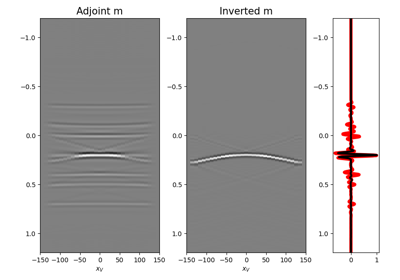# pylops.waveeqprocessing.MDD#

pylops.waveeqprocessing.MDD(G, d, dt=0.004, dr=1.0, nfmax=None, wav=None, twosided=True, causality_precond=False, adjoint=False, psf=False, dottest=False, saveGt=True, add_negative=True, smooth_precond=0, fftengine='numpy', **kwargs_solver)[source]#

Multi-dimensional deconvolution.

Solve multi-dimensional deconvolution problem using scipy.sparse.linalg.lsqr iterative solver.

Parameters
Gnumpy.ndarray

Multi-dimensional convolution kernel in time domain of size $$[n_s \times n_r \times n_t]$$ for twosided=False or twosided=True and add_negative=True (with only positive times) or size $$[n_s \times n_r \times 2n_t-1]$$ for twosided=True and add_negative=False (with both positive and negative times)

dnumpy.ndarray

Data in time domain $$[n_s \,(\times n_{vs}) \times n_t]$$ if twosided=False or twosided=True and add_negative=True (with only positive times) or size $$[n_s \,(\times n_{vs}) \times 2n_t-1]$$ if twosided=True

dtfloat, optional

Sampling of time integration axis

drfloat, optional

nfmaxint, optional

Index of max frequency to include in deconvolution process

wavnumpy.ndarray, optional

Wavelet to convolve to the inverted model and psf (must be centered around its index in the middle of the array). If None, the outputs of the inversion are returned directly.

twosidedbool, optional

MDC operator and data both negative and positive time (True) or only positive (False)

add_negativebool, optional

Add negative side to MDC operator and data (True) or not (False)- operator and data are already provided with both positive and negative sides. To be used only with twosided=True.

causality_precondbool, optional

Apply causality mask (True) or not (False)

smooth_precondint, optional

Lenght of smoothing to apply to causality preconditioner

adjointbool, optional

psfbool, optional

Compute and return Point Spread Function (PSF) and its inverse

dottestbool, optional

Apply dot-test

saveGtbool, optional

Save G and G.H to speed up the computation of adjoint of pylops.signalprocessing.Fredholm1 (True) or create G.H on-the-fly (False) Note that saveGt=True will be faster but double the amount of required memory

fftenginestr, optional

Engine used for fft computation (numpy, scipy or fftw)

**kwargs_solver

Arbitrary keyword arguments for chosen solver (scipy.sparse.linalg.cg and pylops.optimization.solver.cg are used as default for numpy and cupy data, respectively)

Returns
minvnumpy.ndarray

Inverted model of size $$[n_r \,(\times n_{vs}) \times n_t]$$ for twosided=False or $$[n_r \,(\times n_vs) \times 2n_t-1]$$ for twosided=True

madjnumpy.ndarray

Adjoint model of size $$[n_r \,(\times n_{vs}) \times n_t]$$ for twosided=False or $$[n_r \,(\times n_r) \times 2n_t-1]$$ for twosided=True

psfinvnumpy.ndarray

Inverted psf of size $$[n_r \times n_r \times n_t]$$ for twosided=False or $$[n_r \times n_r \times 2n_t-1]$$ for twosided=True

psfadjnumpy.ndarray

Adjoint psf of size $$[n_r \times n_r \times n_t]$$ for twosided=False or $$[n_r \times n_r \times 2n_t-1]$$ for twosided=True

MDC

Multi-dimensional convolution

Notes

Multi-dimensional deconvolution (MDD) is a mathematical ill-solved problem, well-known in the image processing and geophysical community .

MDD aims at removing the effects of a Multi-dimensional Convolution (MDC) kernel or the so-called blurring operator or point-spread function (PSF) from a given data. It can be written as

$\mathbf{d}= \mathbf{D} \mathbf{m}$

or, equivalently, by means of its normal equation

$\mathbf{m}= (\mathbf{D}^H\mathbf{D})^{-1} \mathbf{D}^H\mathbf{d}$

where $$\mathbf{D}^H\mathbf{D}$$ is the PSF.

1

Wapenaar, K., van der Neut, J., Ruigrok, E., Draganov, D., Hunziker, J., Slob, E., Thorbecke, J., and Snieder, R., “Seismic interferometry by crosscorrelation and by multi-dimensional deconvolution: a systematic comparison”, Geophyscial Journal International, vol. 185, pp. 1335-1364. 2011.

## Examples using pylops.waveeqprocessing.MDD#09. Multi-Dimensional Deconvolution

09. Multi-Dimensional Deconvolution